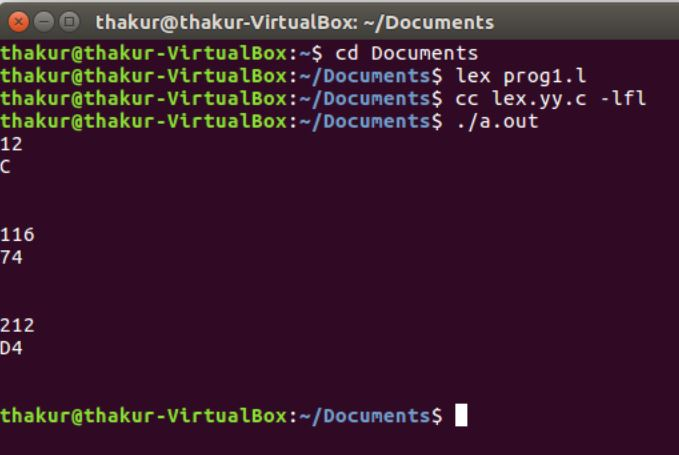Skip to content
Related Articles
Lex program for Decimal to Hexadecimal Conversion
• Last Updated : 30 Apr, 2019

Problem: Write a Lex program for Decimal to Hexadecimal conversion.

Explanation:
Lex reads an input stream specifying the lexical analyzer and outputs source code implementing the lexer in the C programming language. The function yylex() is the main flex function which runs the Rule Section.

Prerequisite: Flex (Fast lexical Analyzer Generator)

Examples:

```Input: 12
Output: C

Input: 116
Output: 74

Input: 55
Output: 37

Input: 212
Output: D4 ```

Implementation:

 `/* Lex program for decimal to hexadecimal conversion */`` ` `%{``    ``/* Definition section */``    ``#include``    ``int` `num, r, digit=0, count, pcount=0, i;``    ``char` `a;``%}`` ` `DIGIT [0-9]``/* Rule Section */``%%`` ` `{DIGIT}+ { num=``atoi``(yytext);`` ` `        ``while``(num!=0)``        ``{`` ` `            ``r=num%16;``            ``digit=``'0'``+r;``            ``if``(digit>``'9'``)``            ``digit+=7;``            ``a[count++]=digit;``            ``num=num/16;`` ` `        ``}`` ` `        ``for``(i=count-1;i>=pcount;--i)``                ``printf``(``"%c"``, a[i]);``                ``pcount=count;``        ``}`` ` `.|\n    ECHO;``        ` `%%`` ` `// driver code``int` `main()``{``    ``yylex();``    ``return` `0;``}      `

Output:Attention reader! Don’t stop learning now. Get hold of all the important CS Theory concepts for SDE interviews with the CS Theory Course at a student-friendly price and become industry ready.

My Personal Notes arrow_drop_up